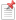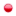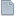###Author Topic: MC624 Serial Communication Protocol

February 18, 2023, 12:10:51 PM#### JPK

• Hero Member
•• Posts: 855
• Karma: +26/-0##### MC624 Serial Communication Protocol
For those interested, this is the communication protocol used by the MC624.
The data are sent via the USB connector pair at the back of the unit.

It is possible to drive the MC624 from the usb connector. We use an USB connector for convenience but the signal going through is actually a standard serial asynchronous RS232, 8 bits, 9600 Bds, port interfaced to RS485.
When the unit is set to master, the serial link is an output.
When the unit is set to slave, the serial link is an input.

MC624 Protocol :
There are 3 commands made of one byte each:

Volume command:Bit 7 bit 6 = 00
The volume is binary coded from 0 (00000) to 63 (11111)

Input/Output select command:Bit 7 bit 6 = 01
The SUB state is coded in bit 5. 0 = OFF, 1 = ON
The output channel is binary coded in bits 3 & 4, from 00 (channel 1) to 11 (channel 4)
The input channel is binary coded in bits 0, 1 & 2, from 000 (channel 1) to 101 (channel 6)
You must send both the IN and OUT numbers in each command.

Functions command:Bit 7 bit 6 = 10
The MUTE Left state is coded in bit 5. 0 = OFF, 1 = Mute left
The MUTE Right state is coded in bit 4. 0 = OFF, 1 = Mute Right
The MONO state is coded in bit 3. 0 = Stereo, 1 = Mono
The DIFF state is coded in bit 2. 0 = Normal, 1 = Diff
The DIM state is coded in bit 0. 0 = Normal, 1 = Dim
Bit 1 is not used.
You must send all the 5 states in each function command.

« Last Edit: February 18, 2023, 12:22:52 PM by JPK »
JPK